專利授權區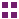專利授權區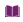專利摘要(中)
A method of training an N-bit neural network (N≧2), is proposed to include: providing the N-bit neural network that includes a plurality of weights to be trained, each of the weights being composed of N bits that respectively correspond to N bit orders which are divided into multiple bit order groups, wherein the bits of the weights are divided, based on the bit orders to which the bits of the weights correspond, into multiple bit groups that respectively correspond to the bit order groups; and determining the weights for the N-bit neural network by training the bit groups one by one.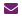聯絡資訊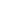我有興趣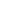BACK